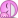# Dx before the f(x) in integrals

Mentor
2022 Award
Why would it be called that?
Wasn't he the first who came up with this idea?

Wasn't he the first who came up with this idea?
Aristoteles! Do you mean Archimedes?

Mentor
2022 Award
Aristoteles! Do you mean Archimedes?
Sure. How embarrassing ...

Gold Member
But if you are going to use ##\int df=f## and ##\int dx = x## in that line, then you may as well say that ##\int fdx=\int dxf=xf=fx## with the appropriate ##\cong##'s.
Not if you are a physicist with an intuitive feeling for limitations of any heuristic rules.Sure.
That is a good question on its own.

Gold Member
•fresh_42
Staff Emeritus
Which doesn't say anything about the notation ##\int fdx##.

But it does seem to write the Lebesgue integral in terms of infinitesimals.

But it does seem to write the Lebesgue integral in terms of infinitesimals.
But those infinitesimals are for another function. The point was to be able to make sense,at least intuitively, of ##\int dxf(x)## as an infinite sum of infinitesimals.

Gold Member
Would it be correct to say that both Riemann and Lebesque integrals are just special cases of computing the area
$$\int\int dxdy$$
of a region on a 2-dimensional plane?

And what do you mean by area? The Lebesgue measure of the set? The integral you wrote down? What exactly is that integral?

Staff Emeritus
And what do you mean by area? The Lebesgue measure of the set? The integral you wrote down? What exactly is that integral?

Let ##f## be a function from reals to reals (for simplicity, let it be nonnegative). Let ##S(A,B)## be the set of points ##(x,y)## such that ##A \leq x \leq B## and ##0 \leq y \leq f(x)##. Then if the area of set ##S(A,B)## is defined, it will be equal to ##\int_A^B f(x) dx##. Right? By "area" I mean a measure on ##R^2## such that every set of the form ##\{ (x,y) | x_0 \leq x \leq x_1 \wedge y_0 \leq y \leq y_1\}## has measure ##|(x_1 - x_0) \cdot (y_1 - y_0)|##

Let ##f## be a function from reals to reals (for simplicity, let it be nonnegative). Let ##S(A,B)## be the set of points ##(x,y)## such that ##A \leq x \leq B## and ##0 \leq y \leq f(x)##. Then if the area of set ##S(A,B)## is defined, it will be equal to ##\int_A^B f(x) dx##. Right? By "area" I mean a measure on ##R^2## such that every set of the form ##\{ (x,y) | x_0 \leq x \leq x_1 \wedge y_0 \leq y \leq y_1\}## has measure ##|(x_1 - x_0) \cdot (y_1 - y_0)|##
May be, it depends on the unstated assumptions. Say you take area to mean the Lebesgue measure in the plane. It satisfies your requirement for area. Take a function ##f(x)## so that ##S(A,B)## is Lebesgue measurable and ##f(x)## is not Riemann integrable. Then if the integral you wrote is the Riemann integral, then the area is not it, the integral doesn't exists. I assume that is not what you mean, but you didn't say anything about the relationship between the measure in the plane and the integral on the line in you statement. But let's say that it is all fine. Then what? Where are you going with this?

Staff Emeritus
But let's say that it is all fine. Then what? Where are you going with this?

I was just trying to answer the question "What do you mean by area"?$$\int\int dxdy$$